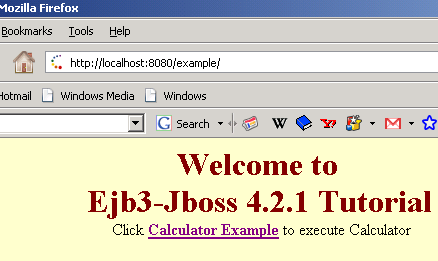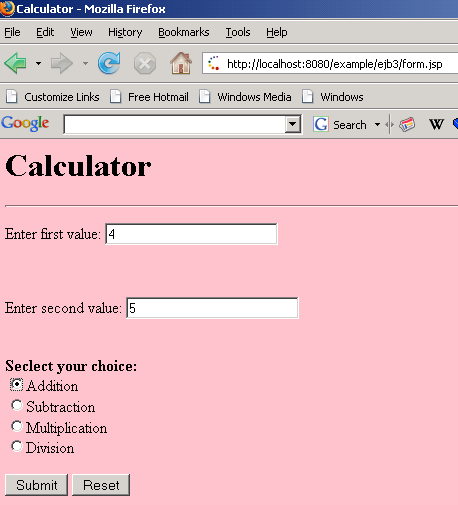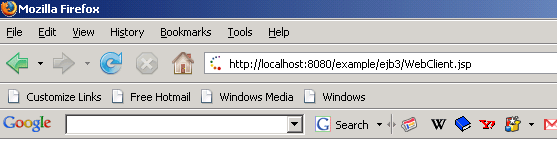# Stateless Session Bean Example

Tutorials# Stateless Session Bean Example

In this part of Enterprise Session Beans, you will learn how to develop, deploy, and run a simple Java EE application named example using stateless session bean.

In this part of Enterprise Session Beans, you will learn how to develop, deploy, and run a simple Java EE application named example using stateless session bean.

# Stateless Session Bean Example

In this part of Enterprise Session Beans, you will learn how to develop, deploy, and run a simple Java EE application named example using stateless session bean. The purpose of example is to performs the mathematical operations such as Addition, Subtraction, Multiplication, and Division.
The example application consists of an enterprise bean, which performs the calculations, and two types of clients: an application client and a web client.

There are following steps that you have to follow to develop a example JEE application.

1. Create the enterprise bean: CalculatorBean

2. Create the web client: WebClient

3. Deploy example onto the server.

4. Using a browser, run the web client.

I. Creating the enterprise bean:

The enterprise bean in our example is a stateless session bean called CalculatorBean. The source code for CalculatorBean is in ?net/roseindia/ejb3/stateless? directory.

Creating CalculatorBean requires these steps:

(i) Coding the bean?s Remote business interface and Enterprise bean class.

(ii) Compiling the source code with the Ant tool.

The business interface defines the business methods that a client can call remotely. The business methods are implemented in the enterprise bean class. The source code for the CalculatorRemote business interface is given below.

 ``` package net.roseindia.ejb3.stateless;``` ``` import java.math.*; import javax.ejb.Remote; import java.lang.annotation.*; @Remote public interface CalculatorRemote {  public float add(float x, float y);  public float subtract(float x, float y);  public float multiply(float x, float y);  public float division(float x, float y); }```

Note that, the @Remote annotation decorating the interface definition. This lets the container know that CalculatorBean will be accessed by remote clients.

II. Coding the Enterprise Bean Class

The enterprise bean class for this example is called CalculatorBean. This class implements the four business methods (add, subtract, multiply, division) that are defined in the CalculatorRemote  business interface. The source code for the CalculatorBean class is given below.

 ``` package net.roseindia.ejb3.stateless; import java.math.*; import javax.ejb.Stateless; import javax.ejb.Remote;   @Stateless(name="CalculatorBean") @Remote(CalculatorRemote.class)   public class CalculatorBean implements CalculatorRemote{   public float add(float x, float y){    return x + y;  }  public float subtract(float x, float y){   return x - y;  }   public float multiply(float x, float y){   return x * y;  }  public float division(float x, float y){  return x / y;  } }```

Note that, the @Stateless annotation decorating the enterprise bean class. This lets the container know that CalculatorBean is a stateless session bean.

Compiling and Packaging the example Example

Now you are ready to compile the remote business interface (CalculatorRemote.java), the enterprise bean class (CalculatorBean.java) and the application client (CalculatorClient.java), then package the compiled classes into an enterprise bean JAR.

II. Creating the calculator Web Client

The web client is contained in the JSP page "WebClient.jsp". A JSP page is a text-based document that contains JSP elements, which construct dynamic content, and static template data, expressed in any text-based format such as HTML, WML, and XML.

The source code for the ?form.jsp? is given below.

     Calculator

Calculator

Enter first value:

Enter second value:

Subtraction
Multiplication
Division

The following statements given below in ?WebClient.jsp? are used for locating the business interface, creating an enterprise bean instance, and invoking a business method.

 InitialContext ic = new InitialContext(); CalculatorRemote calculator = (CalculatorRemote)ic.lookup("example/CalculatorBean/remote");

The classes needed by the client are declared using a JSP page directive (enclosed within the <%@ %> characters). Because locating the business interface and creating the enterprise bean are performed only once, this code appears in a JSP declaration (enclosed within the <%! %> characters) that contains the initialization method, jspInit, of the JSP page. A scriptlet (enclosed within the <% %> characters) retrieves the parameters from the request and converts it to a Float object. Finally, a JSP scriptlet invokes the enterprise bean?s business methods, and JSP expressions (enclosed within the <%= %> characters) insert the results into the stream of data returned to the client.

The full source code for the WebClient program is given below.

 ``` <%@ page contentType="text/html; charset=UTF-8" %> <%@ page import="com.javajazzup.examples.ejb3.stateless.*,  javax.naming.*"%>  <%!   private CalculatorRemote calculator = null;   float result=0;   public void jspInit() {   try {      InitialContext ic = new InitialContext();      calculator = (CalculatorRemote) ic   .lookup("example/CalculatorBean/remote");   System.out.println("Loaded Calculator Bean"); //CalculatorBean   } catch (Exception ex) {   System.out.println("Error:"+   ex.getMessage());   }   }   public void jspDestroy() {   calculator = null;   } %>   <%   try {   String s1 = request.getParameter("num1");   String s2 = request.getParameter("num2");   String s3 = request.getParameter("group1"); System.out.println(s3);   if ( s1 != null && s2 != null ) {   Float num1  = new Float(s1);   Float num2  = new Float(s2);      if(s3.equals("add"))   result=calculator.add(num1.floatValue(), num2.floatValue());   else if(s3.equals("sub"))   result=calculator.subtract(num1.floatValue(), num2.floatValue());   else if(s3.equals("multi"))   result=calculator.multiply(num1.floatValue(), num2.floatValue());   else   result=calculator.division(num1.floatValue(), num2.floatValue());     %>

The result is: <%= result %>

<%   }   }// end of try   catch (Exception e) {   e.printStackTrace ();  //result = "Not valid";   }   %> ```

Note: The Application Server automatically compiles web clients that are JSP pages. If the web client were a servlet, you would have to compile it.

The source code for the ?index.jsp? is given below that will actual call the client-design form.

 <%@page language="java" %> Ejb3 Stateless Tutorial

Welcome to
Ejb3-Jboss 4.2.0 Tutorial
Click Calculator Example to execute Calculator

III. Deploy example onto the server

To deploy the created example application we are going to use Jboss 4.2.0 Application Server about which you have read in the previous section of this Javajazzup issue. So you first need to download the following tools to deploy this application.

• JDK 1.5 or Higher
•  apache-ant-1.7.0
• JBoss 4.2.0

Do the following steps to deploy the example application:

(ii)  Create the essential deployment descriptor .xml files.

build.xml



Put this file in the base (stateless\code)directory.

Application.xml

   Stateless Session Bean Example         example.war     /example           example.jar

Put this file in the Stateless\code\deploymentdescriptors\ear directory.

web.xml



Put this file in the Stateless\code\deploymentdescriptors\web directory.
Put all .jsp files in the Stateless\code\web directory.
Put all .java files in the Stateless\code\src directory.

(iii) Start command prompt, and go to the Stateless\code directory. Then type the command as:
C:\Stateless\code>ant build.xml

The Ant tool will deploy the example.ear file to the jboss-4.2.0.GA\server\default\deploy directory.

V. Running the example Web Client

Open the web browser and type the following URL to run the application:

http://localhost:8080/exampleClick at the given link as Calculator Example:Give values to the textbox and choose the desire option button as Addition then clicks the Submit button to get the result.The result is: 9.0